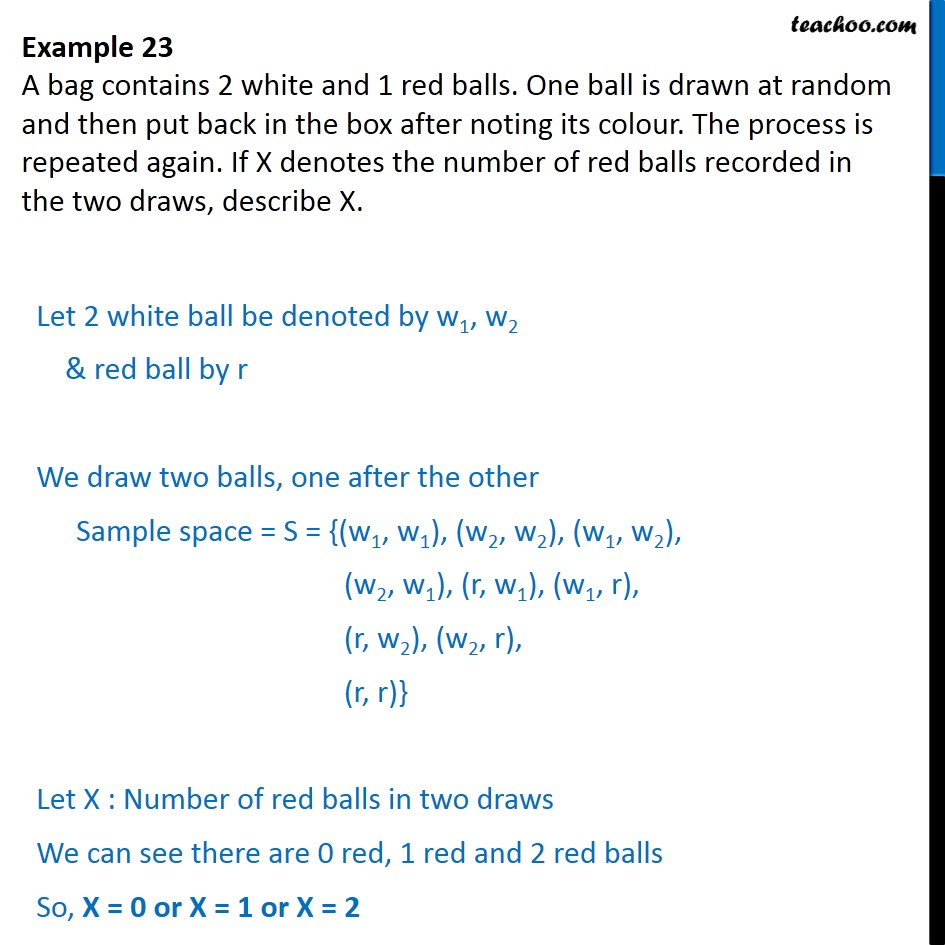Random Variable - Defination

Chapter 13 Class 12 Probability (Term 2)
Concept wise### Transcript

Example 23 A bag contains 2 white and 1 red balls. One ball is drawn at random and then put back in the box after noting its colour. The process is repeated again. If X denotes the number of red balls recorded in the two draws, describe X. Let 2 white ball be denoted by w1, w2 & red ball by r We draw two balls, one after the other Sample space = S = {(w1, w1), (w2, w2), (w1, w2), (w2, w1), (r, w1), (w1, r), (r, w2), (w2, r), (r, r)} Let X : Number of red balls in two draws We can see there are 0 red, 1 red and 2 red balls So, X = 0 or X = 1 or X = 2